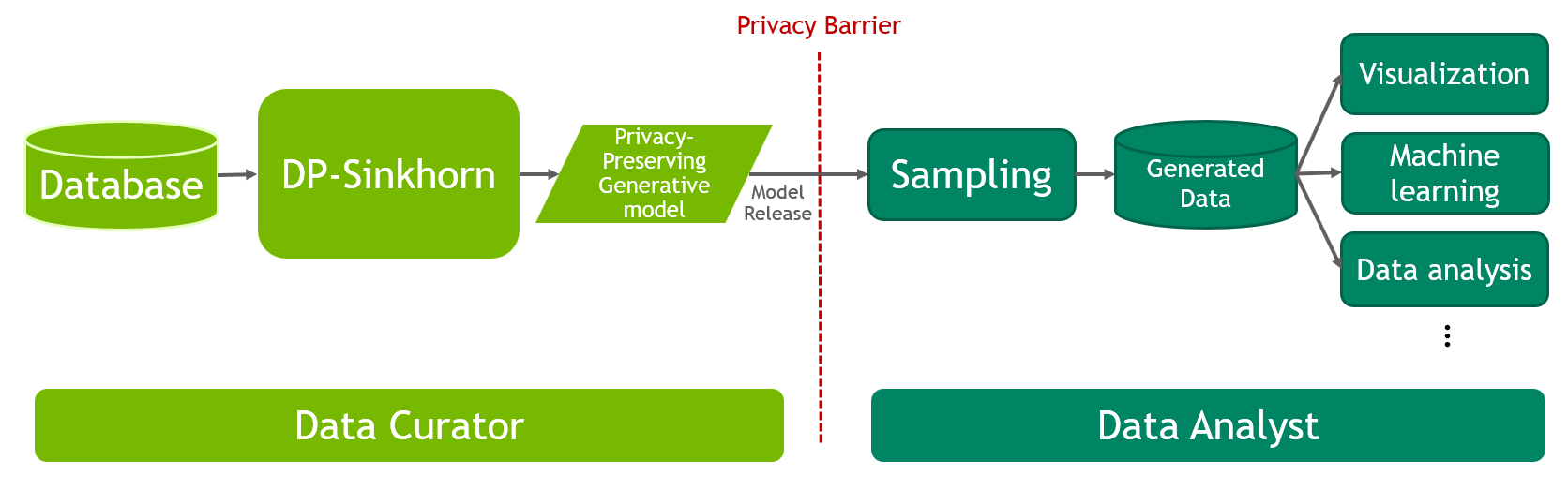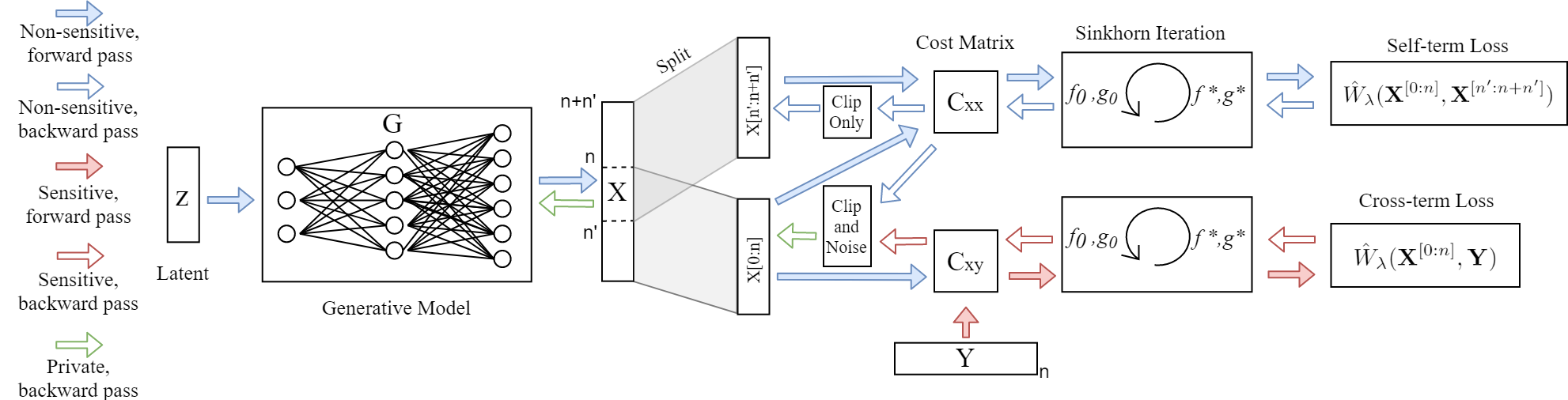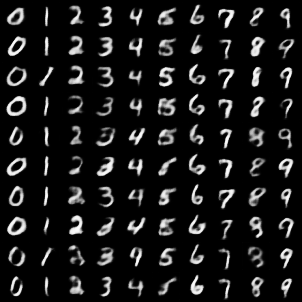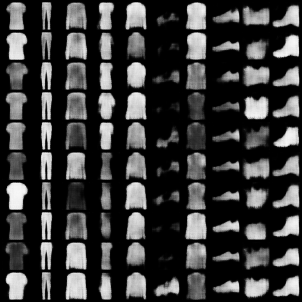# Don't Generate Me: Training Differentially Private Generative Models with Sinkhorn Divergence

• 1University of Toronto
• 2Vector Institute
• 3University of Waterloo
• 4NVIDIA

NeurIPS 2021

[Paper PDF] [Supplementary Materials] [Arxiv] [Code]We propose DP-Sinkhorn, a method for privacy-preserving generative modeling. With DP-Sinkhorn, private data held by data curators can be shared to analysts for data-driven downstream tasks while preserving the privacy of the data. First, the curator uses DP-Sinkhorn to train a privacy-preserving generative model. Then, this model is shared to analysts, who sample the model for generated data. The generated data is similar to the private data, yet does not reveal details about individual data points. Various tasks can be performed on the generated data in place of the private data.

## Abstract

Although machine learning models trained on massive data have led to breakthroughs in several areas, their deployment in privacy-sensitive domains remains limited due to restricted access to data. Generative models trained with privacy constraints on private data can sidestep this challenge, providing indirect access to private data instead. We propose DP-Sinkhorn, a novel optimal transport-based generative method for learning data distributions from private data with differential privacy. DP-Sinkhorn minimizes the Sinkhorn divergence, a computationally efficient approximation to the exact optimal transport distance, between the model and data in a differentially private manner and uses a novel technique for controlling the bias-variance trade-off of gradient estimates. Unlike existing approaches for training differentially private generative models, which are mostly based on generative adversarial networks, we do not rely on adversarial objectives, which are notoriously difficult to optimize, especially in the presence of noise imposed by privacy constraints. Hence, DP-Sinkhorn is easy to train and deploy. Experimentally, we improve upon the state-of-the-art on multiple image modeling benchmarks and show differentially private synthesis of informative RGB images.

## Motivation

Differential privacy (DP) is a rigorous definition of privacy that quantifies the amount of information leaked by a user, participating in a data release . DP was originally designed for answering queries to statistical databases. In a typical setting, a data analyst (party wanting to use data; e.g. a healthcare company) sends a query to a data curator (party in charge of safekeeping the database; e.g. a hospital), who makes the query on the database and replies with a semi-random answer that preserves privacy. Responding to each new query incurs a privacy cost. If the analyst has multiple queries, the curator must subdivide the privacy budget to spend on each query. Once the budget is depleted, the curator can no longer respond to queries, preventing the analyst from performing new, unanticipated tasks with the database. To sidestep this challenge, generative models can be applied as a general and flexible data-sharing medium: The curator trains a generative model with DP guarantees, and releases the model to analysts. The analysts then generate data from this model, which can be used for any downstream task. In this work, our goal is to learn a generative model while satisfying the constraints of differential privacy.

Differentially private learning of generative models has been studied mostly using generative adversarial networks (GANs). While GANs in the non-private setting can synthesize complex data such as high definition images, their application in the private setting is challenging. This is in part because GANs suffer from training instabilities, which can be exacerbated by adding noise to the network's gradients during training, a common technique to implement DP. Hence, GANs typically require careful hyperparameter tuning and supervision during training to avoid collapsing. This goes against the principle of privacy, where repeated interactions with data need to be avoided. To overcome these issues, we propose a non-adversarial generative learning approach that enjoys stabler convergence, produces higher quality outputs, and is more robust to the choice of hyperparameters.

## Method Overview

We propose DP-Sinkhorn, a novel method to train differentially private generative models using a semi-debiased Sinkhorn loss. DP-Sinkhorn is based on the framework of optimal transport (OT), where the problem of learning a generative model is framed as minimizing the optimal transport distance, a type of Wasserstein distance, between the generator-induced distribution and the real data distribution. The optimal transport distance has many desirable properties as an objective function, but they are difficult to compute due to the optimization problem on the transport plan. The entropy regularized Wasserstein distance  makes computation of the transport plan tractable at the cost of introducing bias:

$$W_{c,\lambda}(\mu, \nu)= \min_{\pi \in \Pi} \int c({x},{y})d\pi({x},{y}) + \lambda \int \log\left( \frac{d\pi({x},{y})}{d\mu({x})d\nu({y})}\right)d\pi({x},{y}),$$

where $$\pi(x,y)$$ is the transport plan defined as $$\Pi = \{ \pi(x,y) \in \mathcal{P}(\mathcal{X} \times \mathcal{X})| \int \pi(x, \cdot) d x = \nu, \int \pi(\cdot, y) d y = \mu\}$$, with cost function $$c(x,y)$$, and regularization weight parameter $$\lambda$$.

The Sinkhorn divergence uses auto-correlation terms to reduce the entropic bias introduced by ERWD with respect to the exact Wasserstein distance . Empirically computing the Sinkhorn divergence exhibits a bias-variance trade-off. One option is to compute it with a single batch of real data $$X$$ and a single batch of generated data $$Y$$ as:

$$\hat{S}_{c, \lambda}(X, Y) = 2 \hat{W}_\lambda (X, Y) -\hat{W}_\lambda (X, X) - \hat{W}_\lambda (Y, Y).$$

This is a biased estimator as it under-estimates the magnitudes of the two auto-correlation terms. Alternatively, if we independently draw two batches of real and generated data, we obtain an unbiased estimator:

$$\hat{S}_{c, \lambda}(X, Y, X', Y') = 2\hat{W}_\lambda (X, Y) - \hat{W}_\lambda (X, X') - \hat{W}_\lambda (Y, Y').$$

While unbiased, this formulation adds variance to the mini-batched gradients which can be harmful to training. Note that the last term in these two equations affects solely the real data, which is not a function of the generator. Hence, this term has no impact on the learning problem. We design a semi-debiased formulation that controls the bias-variance tradeoff of Sinkhorn divergence. Specifically, the semi-debiased Sinkhorn loss partially re-samples the first batch to create the second batch. The amount of variance can be controlled by adjusting the portion of the batch that is re-sampled. The semi-debiased Sinkhorn loss is formally defined as below.

Definition (Semi-debiased Sinkhorn Loss) For a mixture fraction $$p \in [0,1]$$ and natural number $$n$$, $$n' = floor(n \times p)$$. Given $$n+n'$$ generated samples $$X \in \mathcal{X}^{n+n'}$$ and $$m$$ real samples $$Y \in \mathcal{X}^{m}$$, the semi-debiased Sinkhorn loss is defined as:

$$\hat{S}_{c, \lambda, p}(X, Y) = 2 \hat{W}_\lambda (X^{[0:n]}, Y) -\hat{W}_\lambda (X^{[0:n]}, X^{[n':n+n']})$$

This semi-debiased loss can be viewed as interpolating between the biased Sinkhorn loss ($$p=0$$) and the fully unbiased Sinkhorn loss ($$p=1$$).Flow diagram of DP-Sinkhorn for a single training iteration

We use the semi-debiased Sinkhorn loss to train generative models. In each iteration, we first generate synthetic data and sample real data, with the synthetic data split into two batches to perform debiasing. An element-wise cost function that measures the difference between pairs of examples is applied on each element of synthetic and real data to form the cost matrix. Then, the semi-debiasing Sinkhorn divergence is calculated on these batches. Privacy protection is achieved by applying the Gaussian mechanism (a well-known method for adding noise to achieve DP) to gradients of the Sinkhorn loss w.r.t generated images.

## Experimental ResultsWe evaluate DP-Sinkhorn on popular benchmarks of MNIST and Fashion MNIST for privacy-preserving generative modelling. Above are synthetic images produced by DP-Sinkhorn using a privacy budget of ($$10, 10^{-5}$$)-DP. Images are generated with class conditioning. To measure the utility of generated images, we train a classifier on synthetic data, and evaluate it on held-out real data. DP-Sinkhorn achieves state-of-the-art performance in both image quality and utility among differentially private generative models.We also experiment with DP-Sinkhorn on RGB images. Here, we use CelebA downsampled to 32x32 as real data, and consider the task of gender conditional generation. DP-Sinkhorn is able to produce informative synthetic data for downstream gender classification despite the added image complexity. Images generated by DP-Sinkhorn resemble blurry faces, while existing baselines cannot produce data with reasonable appearance.

## Citation

For feedback and questions please reach out to Tianshi Cao and Karsten Kreis. If you find this work useful for your research, please consider citing it as:

@inproceedings{Cao2021DPSinkhorn,
title = {Don't Generate Me: Training Differentially Private Generative Models with Sinkhorn Divergence},
author = {Tianshi Cao and Alex Bie and Arash Vahdat and Sanja Fidler and Karsten Kreis},
booktitle = {Advances in Neural Information Processing Systems (NeurIPS)},
year = {2021}
}


### References

 C. Dwork and A. Roth, “The Algorithmic Foundations of Differential Privacy,” Foundations and Trends in Theoretical Computer Science, vol. 9, p. 211–407, Aug. 2014.
 G. Peyré and M. Cuturi, “Computational Optimal Transport,” Foundations and Trends in Machine Learning, vol. 11, no. 5-6, pp. 355–607, 2019.
 J. Feydy, T. Séjourné, F.-X. Vialard, S.-i. Amari, A. Trouvé, and G. Peyré, “Interpolating between optimal transport and MMD using sinkhorn divergences,” in The 22nd International Conference on Artificial Intelligence and Statistics, pp. 2681–2690, 2019.